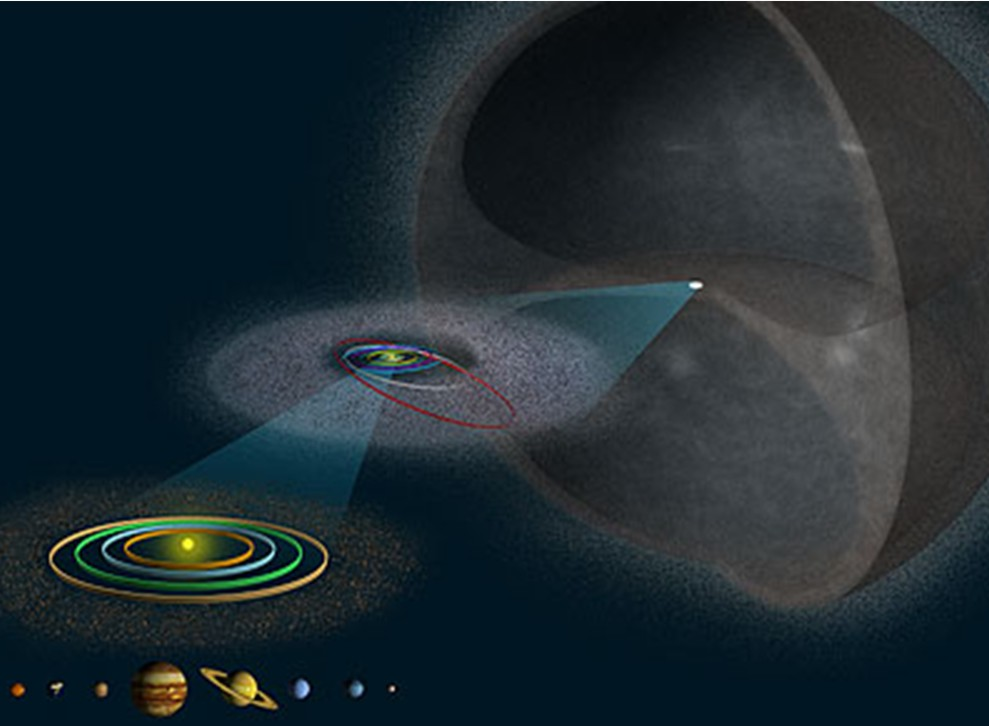# Invariable Plane

In Solar System by Brian Koberlein1 Comment

One aspect of our solar system is that it is essentially a closed system.  The stars are so widely separated that it would be rare for a stray asteroid or other object from beyond the Oort cloud to enter our solar system.  This means that nearly all the rocky material orbiting the Sun now was also part of the solar system billions of years ago.  This has a few consequences, one of which is the tendency for the solar system to lie in a plane.

With a closed system, certain things are constant and unchanging.  In physics, we say they are conserved.  One of these conserved things is known as angular momentum.  In simple terms, angular momentum is a measure of the amount of rotation of a system, but in actuality it is a bit more complicated.  Angular momentum not only works for rotating bodies such as the Earth rotating on its axis, but also for collections of objects moving in different directions. For example, each of the planets orbiting the Sun has an angular momentum about the Sun.

The orbits of all the planets don’t lie within a single plane, but they are relatively close.  However, if you add the angular momenta of all the planets, (and all the asteroids, Kuiper belt, etc) you get a total angular momentum of the solar system.  Most of this angular momentum is due to the large outer planets.  Together they account for about 98% of the total angular momentum of the solar system.

This total angular momentum can be defined by a plane known as the invariable plane.  It’s invariable because the total angular momentum of the solar system is constant.  The orbits of individual planets can change due to gravitational interactions, but the invariable plane can’t change. If the Earth’s orbit shifts relative to the invariable plane due to a gravitational interaction with Jupiter, then Jupiter’s orbit must also shift.  Individual planets can gain or lose angular momentum, but they do so by taking it from or giving it to other planets.

Conservation of momentum also explains why the solar system (and other rotating systems like galaxies) tend to be planar.  Imagine an early solar system where all the different matter is moving around the proto-Sun in all directions.  All this higgledy-piggledy motion, with everything from the largest proto-planet to the smallest dust grain, has some total angular momentum.  In principle you could add up the angular momentum of every object in the early solar system, and the total would be defined by the invariable plane. As objects collide and merge, the angular momentum of the new object is equal to the sum of the originals.  As more and more objects collide, the sum of the larger and larger objects will tend toward the total angular momentum of the system. So as the planets formed, they tended to form along the invariable plane of the solar system.

This doesn’t mean that everything will tend toward the same plane.  There are, for example, comets that have an orbital plane radically different from the invariable plane.  But if you take the average of all these comets, this average is close to the invariable plane. There is also the Oort cloud on the outer edge of our solar system, which is distributed evenly around the Sun, and not in a plane at all.

But for the main planets, the invariable plane marks the plane of angular momentum that was there when the solar system began. And through the physics of  mutual interaction drew the planets close to it.

1.